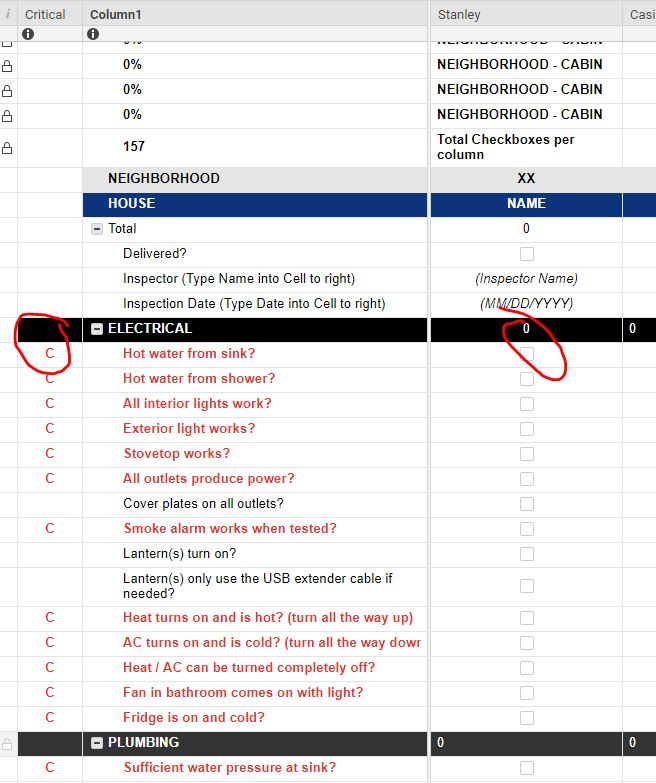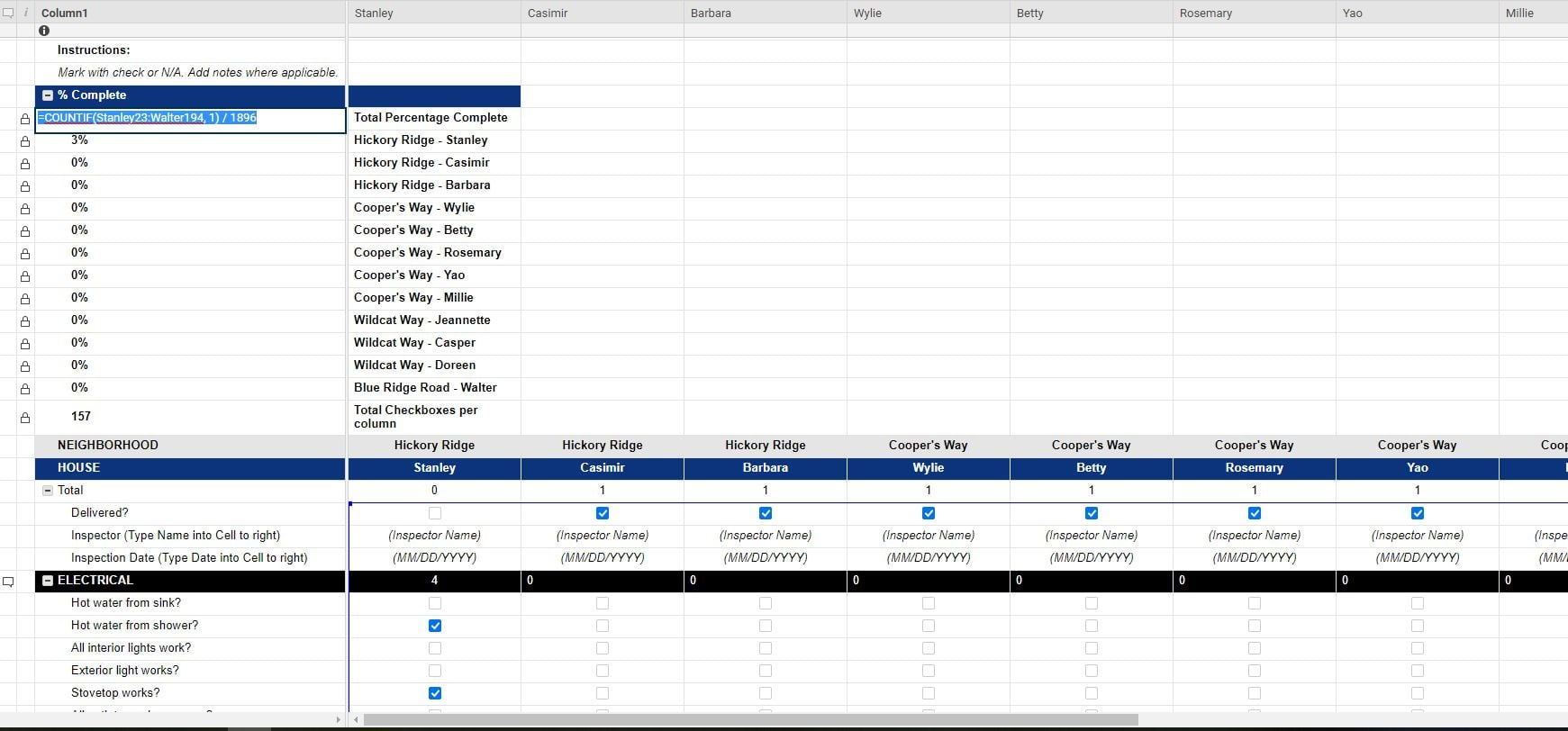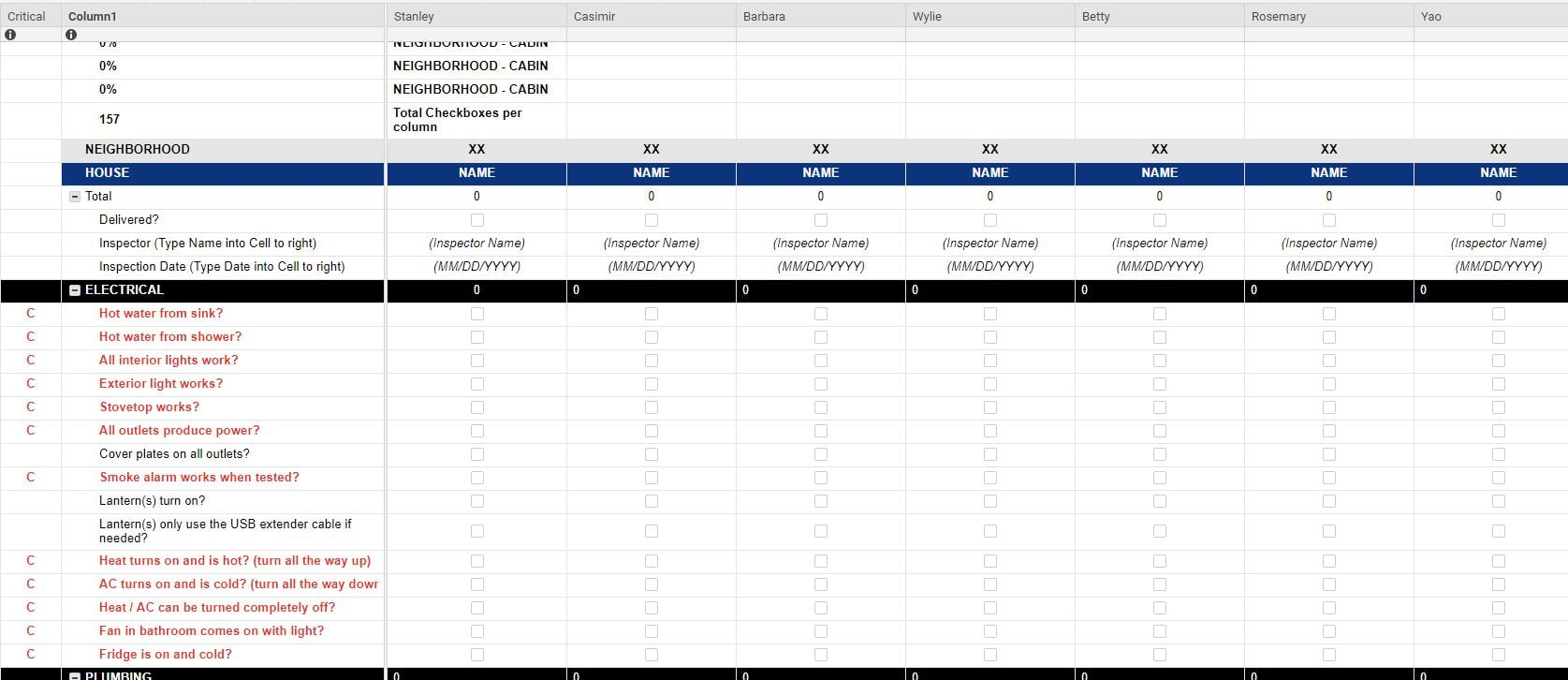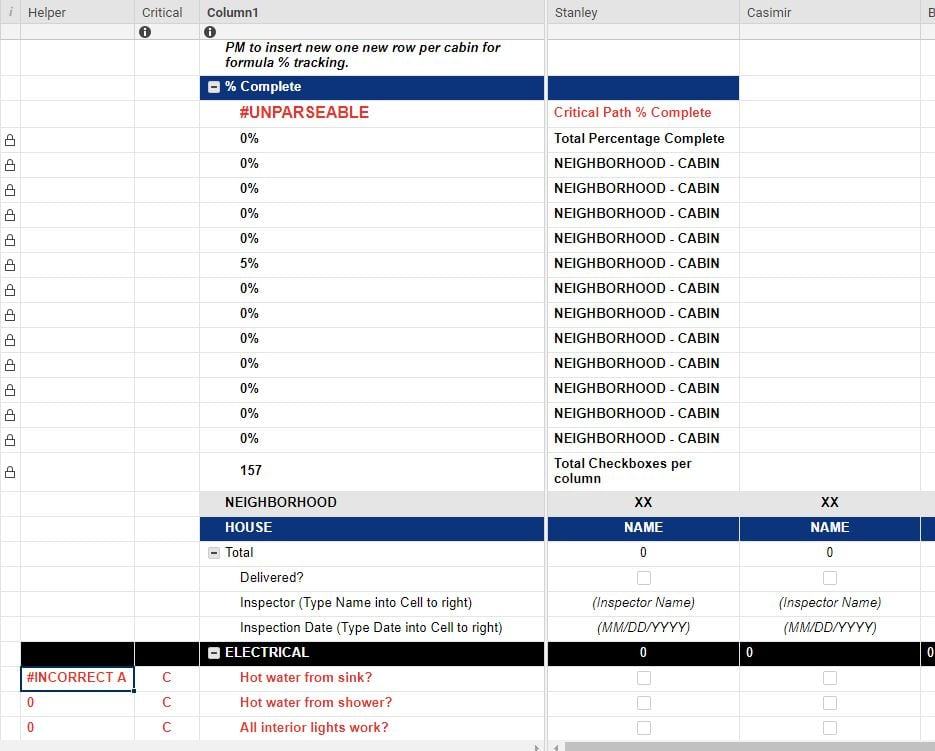# Formula -weighted for specific criteria

I have a complicated request. I want to create a formula that will provide a % when a row is designated as a "critical path" item. See screenshot. As a box is checked in the column for each cabin name, I want to get the % to update for those critical checked boxes.

Is there a way to do this? I already have a formula that takes into account the entire sheet, all checkboxes and the formula of percent complete for all boxes checked. But want to separately tally the critical ones too.

Thank you.• Jenn - For the entire sheet or just the section?

• This should only require a slight variation of the formula you are currently using. Can you post your current formula that generates the % complete for the checked boxes?

• The formula I need would be for all the critical path items in the entire sheet, which includes multiple columns & rows.

=COUNTIF(Stanley23:Walter194, 1) / 1896

This is the formula for the entire sheet, to get the % complete of cabins.

But now, I need it to capture the critical path items (noted with a 'C', in red) as a separate % complete.• Ok. Try switching over to a COUNTIFS for some additional criteria and all we will need to do is add in to look for a "C".

=COUNTIFS(Stanley23:Walter194, 1, Critical23:Critical194, @cell = "C") / ####

• This did not work. It is returning an error #UNPARSEABLE

Below is the formula I used:

=COUNTIFS(Stanley31:Walter197, 1, Critical31:Critical197, @cell='"C") /1884

Any help is appreciated.

Thanks,

Jen

• You have an extra apostrophe before the C. I am not sure if the quote is first or second because all 3 marks look the same, but that may be the issue.

• Thanks, I fixed that and now it is saying #INCORRECT ARGUMENT SET

• That's what I was afraid of. It's because the ranges aren't the same size. I am going to suggest a helper column. Text/Number type with the following formula.

=COUNTIFS(Stanley@row:Walter@row, 1, Critical@row, @cell = "C")

You would then use this...

=SUM(Helper 31: Helper197) / 1884

• Where do I put each formula? Which row and column. Sorry, but this is hard to follow.

• Sorry about that. The helper column can go anywhere and can be hidden once you are done setting everything up.

The COUNTIFS formula would go in every row of the helper column.

The SUM formula goes wherever it is you are wanting to display the percentage.

• Still not working. I added the Helper column, and tried to add this formula row by row, and I get an error message.• Ugh. I am so sorry. It's Monday.

In the helper column...

=IF(Critical@row = "C", COUNTIFS(Stanley@row:Walter@row, 1)

• This worked! Thank you so much for figuring this out for me. I greatly appreciate it.

Jen

• Happy to help.Sorry for the initial confusion. I hate Mondays.

## Help Article Resources

Want to practice working with formulas directly in Smartsheet?

Check out the Formula Handbook template!